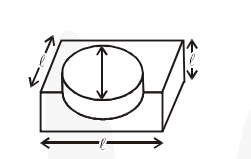# A hemispherical depression is cut out from one face of a cubical wooden block such`
Question:

A hemispherical depression is cut out from one face of a cubical wooden block such that the diameter $\ell$ of the hemisphere is equal to the edge of the cube. Determine the surface area of the remaining solid.

Solution:

Let $\ell$ be the side of the cube.

$\therefore$ The greatest diameter of the hemisphere $=\ell$

$\Rightarrow$ Radius of the hemisphere $=\frac{\ell}{\mathbf{2}}$

$\therefore$ Surface area of hemisphere $=2 \pi \mathrm{r}^{2}$

$=2 \times \pi \times \frac{\ell}{\mathbf{2}} \times \frac{\ell}{\mathbf{2}}=\frac{\pi \ell^{2}}{\mathbf{2}}$Base area of the hemisphere $=\pi\left(\frac{\ell}{2}\right)^{2}=\frac{\pi \ell^{2}}{4}$

Surface area of the cube $=6 \times \ell^{2}=6 \ell^{2}$

$\therefore$ Surface area of the remaining solid

$=6 \ell^{2}+\frac{\pi \ell^{2}}{2}-\frac{\pi \ell^{2}}{4}=\frac{24 \ell^{2}+2 \pi \ell^{2}-\pi \ell^{2}}{4}=\frac{24 \ell^{2}+\pi \ell^{2}}{4}$

$=\frac{\ell^{2}}{4}(24+\pi)$ sq. units.